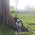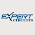# Automatic street light switch using 555 ic

Here is a simple, electronics street light switch using LDR and NE555. The working of this circuit is truly based on light sensing, i.e. automatic turn it on in night (no sunlight available) and turn It off at sunshine (sunlight available).
An electronics street light switch is a switching circuit so, for more detail we can divide this circuit into two section i.e. power supply and switching circuit.

In this power supply section the work of step-down transformer is done by register R1 and further rectification to change into 9.1V dc is by diode D1 and zener diode ZD1. The output voltage across zener diode is further filtered by capacitor C1 and C2.

The another section of street light is switching section built around light-dependent resister LDR1 with the help of transistor T1 through T3 and timer IC NE555 (IC1), where LDR1 is used as sensor of this switching circuit.As in day time the resistance of LDR1 remain low but it is reverse in night time i.e. high resistance is offered by LDR1. For this property of LDR1 the timer IC used in this circuit is as inverter. So, high input at pin 3 is provided by low input at pin 2 and vice-versa. Lastly, this inverter is used to turn street bulb B1 on with the help of triac (triac is activated).

The transistor T1 and T2 is remain cut-off to make pin 4 and pin 8 of IC1 low due to light fall on LDR1 during day time. Due to this transistor T3 is also cut-off and trigger voltage is not received by IC1 through pin 2. As a result the output voltage at pin 3 is low which does not activate triac and the street bulb does not glow.

PARTS LIST

Resistors

R1 = 10 KΩ/10-watt

R2 = 33 KΩ

R3 = 39 KΩ

R4, R6, R7 = 10 KΩ

R5 = 100 Ω

Capacitors

C1, C5 = 0.1 µF

C2 = 1000 µF/25V

C3 = 10 µF/25V

C4 = 0.01 µF

Semiconductors

IC1 = NE555 timer IC

T1, T3 = BC548

T2 = 2N2222

ZD1 = 9.1V/0.5V

D1 = 1N4001

Triac1 = BT136

LED1 = RED color

Miscellaneous

LDR1 = light-dependent resistor

F1 = Fuse, 5A

B1 = 100W/ 230V AC

SW1 = On/off Switch

Blog, Updated at: 10:11

1.Hello!

Thanks for the post. I wanna do it on breadboard but I need to ask some questions if you don't mind.

It's hard to find some components such as "C4 = 0.01 µF" and "R1 = 10 KΩ/10-watt".Would it affect a lot the working of circuit if I use a "10K/11W" resistor instead of 10W resistor. How about the 0.01 µF capacitor? Which capacitor can I use instead without changing the working of this circuit?

1.Hi Ismail...
Thanks for your complements. for C4=0.01 µF you can replace this capacitor with 10nF capacitor . and for R1 = 10 KΩ/10-watt im not sure about 10 KΩ/11-watt resistor replacement. But i think it will not effected to much . so you have to try it.

2.Nice Job thank you!

1.